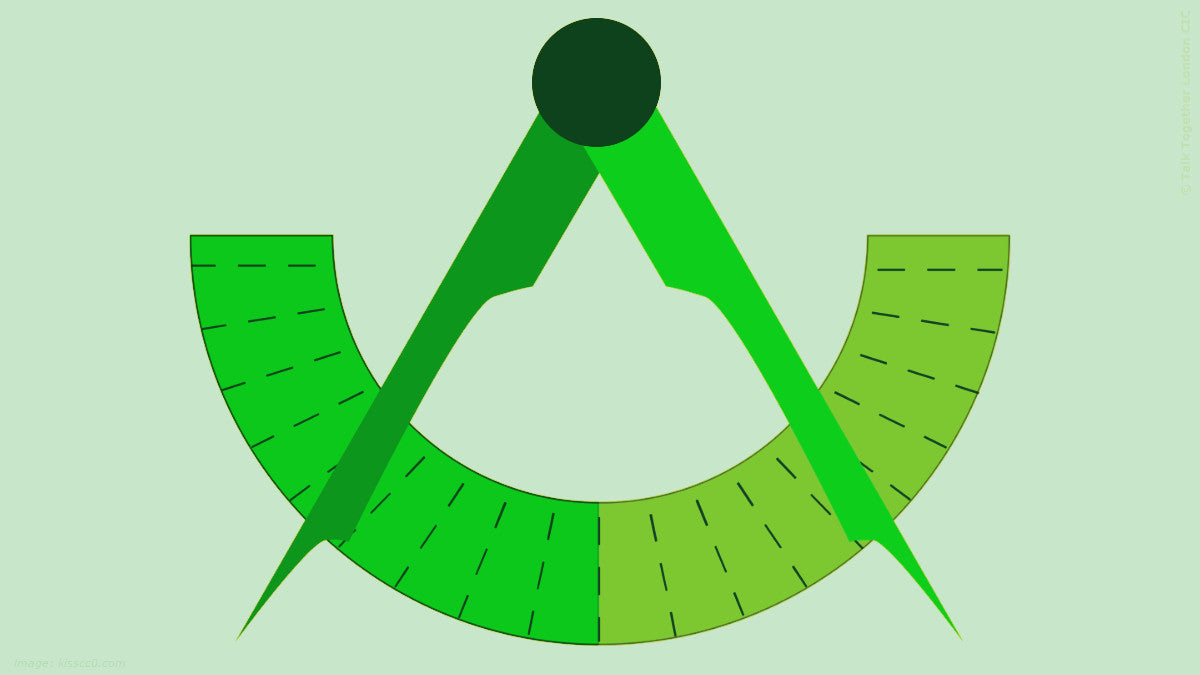# Parents decide: which is more fun - subtraction or division?

When they are helping with homework about subtraction and division, all parents and carers of children will face this question eventually. Which is more difficult: subtraction with borrowing or long division?

In this blog post we look at subtraction and division as examples of how arithmetic is taught to children:

Introduction: are you helping with homework?

The concrete, the pictorial and the abstract

Numbers, physical things and abstract ideas

Preparing for algorithms and rules

The importance of reasons over rules

Conclusion: can talking overcome fear?

### Introduction: are you helping wih homework?

Imagine you are helping with homework to solve the following subtraction and division questions:

4085              1501
- 396         5 ) 7505
3689            - 5
25
- 25
0005
- 5
0

And, you have to explain what happens with borrowing in subtraction and zeros in the dividend when dividing.

At this point many parents' minds probably get flooded with the memorised rules of arithmetic.

And then, it usually looks as if grown-ups are stuck on rules and do not care to understand their meaning – or what is really going on. Probably many teachers emphasized rules rather than reasons in the past, as they still do so now.

Knowing rules and algorithms about the four operations of arithmetic does not guarantee understanding how and why they work. And, if we can not recall the reasons that make them work, then applying the rules does not become a meaningful experience.

How can we ensure that rules and algorithms are learned together with their meaning and children understand the reasons that make them work?

### The concrete, the pictorial and the abstract

In the first three years of primary school - Reception, Year 1 and Year2 - known as Key Stage 1 in the UK, the teaching of arithmetic is very much linked to counting things or measuring things, in other words, dealing with physical objects.

At first, when children begin school, they are tasked with learning how to read and write and at this stage learning arithmetic is about recognizing the numerals 0,1, 2, 3, 4, 5, 6, 7, 8, 9 and counting things.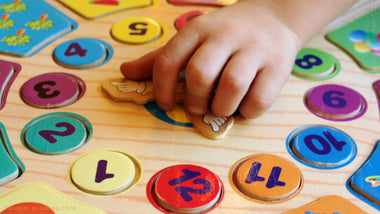But then children are expected to develop two skills simultaneously in Key Stage 2: the ability to think about numbers abstractly and to apply rules and algorithms. In other words, there is a gradual progression from concrete things to pictures and signs about things, and then, finally, from pictures and signs to abstract thinking.

The aim of Key Stage 1 is explained by primary schools as follows:
“Children need to have a secure understanding of number before beginning to calculate. We spend time counting (encouraging accuracy), looking out for numerals and talking about numbers. Children continue to use pictures, marks and informal jottings to help them to calculate.”

After Key Stage 1, children's understanding of arithmetic is expected to get more abstract and they are required to learn rules and how to use them to solve problems.

There is a practical explanation as to why this change takes place: having to deal with numbers that are bigger than 999. In Key Stage 1, children do not work with numbers that have more than two zeros and it is possible to work with these numbers by counting.

In Key Stage 2 children learn about algorithms to manipulate numbers when it is not possible to count in order to find the answer to a problem. They also learn about fractions, decimals and negative numbers – all of which are much more difficult to visualise with real things or pictures of real things.

We can think of a negative number as a debt or a sum of money owed and a minus degree shown on a thermometer, but can you visualise doing the four operations with negative numbers? And, how would you visualise dividing ⅓ by ¼ with real things or pictures of real things?

### Numbers, physical things and abstract ideas

Have you ever considered whether the way literacy is taught to children and the way arithmetic is taught are similar? What is the difference between 'abstract thinking' in arithmetic' and say 'literature'?

In the beginning both literacy and numeracy seem to be dealing with real things. Children are taught with examples from the physical world in Key Stage 1. For example, children will learn how to spell the words ‘umbrella’, 'scarf', 'leaf', 'balloon' and 'hat' in one lesson and count images of these things in another lesson.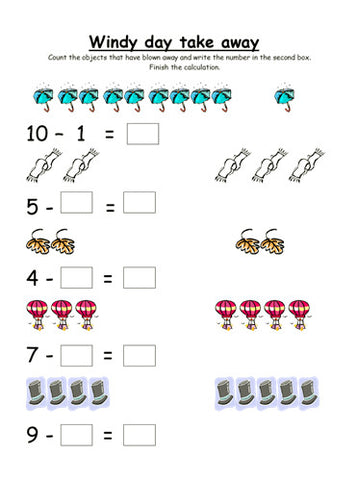Viewing the images of the worksheet titled “Windy day take away”* above, one could conclude that literacy and numeracy are taught similarly because literacy and numeracy both start with learning how to read and write the letters of the alphabet and the numerals zero to ten.

Children who master the alphabet and phonics afterwards advance to spelling grammar and punctuation as they grow older.

What happens after children learn to recognise the numerals from zero to ten and how to count objects in the real world or their images?

Gradually they will begin to think about numbers in a more abstract way, without real world objects or pictures, and they will learn algorithms and rules.

But first they count real things and pictures of things - as in the images of “Windy day take away” - to master the basics of addition, subtraction, multiplication and division.

So, there is a progression from the counting of physical things, to the counting of images and then from the counting of images to an abstract idea of numbers and the relationships between the four operations of arithmetic.

The teaching of arithmetic in Key Stage 1 is supposed to prepare for the development of abstract thinking about numbers in Key Stage 2, together with a meaningful understanding of how and why the rules and algorithms of arithmetic work.

### Counting the big numbers

An algorithm is a set of rules for solving a math problem which, if done properly, will give a correct solution each time.

Algorithms generally involve repeating a series of steps over and over, as in the 'borrowing' and 'carrying' algorithms for subtraction and addition and in the 'long' multiplication and division algorithms.

We can count with our fingers when we are adding 17 and 28, but have you tried to add 234 and 567 by counting? To add 234 and 567 one needs either good mental addition skills or a pen and paper.

Counting doesn't work well with big numbers and so there are more abstract ways of adding that do not require counting of fingers or other things.

Let's look more closely at how one can add 234 and 567. One way is to partition the numbers and add the hundreds, tens and units separately and then combine the sums so: 700 + 90 + 11 = 801.

Another way to add them is to write the numbers down in columns and follow a process called ‘carrying over’:

60+30+10= 100 to carry over
carry over 10
4+7=11
234
+ 567
801

### Preparing for algorithms and rules

The learning of the algorithms and rules has been the core of mathematics education in primary schools in order that students have reliable, accurate methods to do arithmetic by hand, for everyday life and business.

But today’s science and technology demands more from young people than just a knowledge of basic algorithms and rules of mathematics.

Here’s how subtraction is taught in Key Stage 1:Use real objects and encourage children to ‘count how many are left’: I have 6 sweets and eat 3, how many are left? Let’s pretend to eat 3 and count how many are left!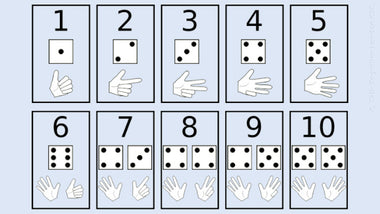Teach children to use their fingers to represent numbers. Practising ‘show me ... fingers’ helps them to use their fingers accurately to support their calculations.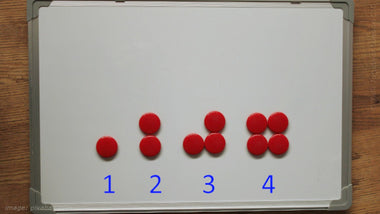Teach the children to count backwards and practise counting down number tracks by jumping and by moving objects. - Can children say one less than any number to 10? - Can they work out two less than a number?Teach children how to use a number line to count backwards. They are also taught to find the difference by ‘counting on’, for example: 85 - 15 = 70. And, they learn how to use empty number lines to help with their calculations.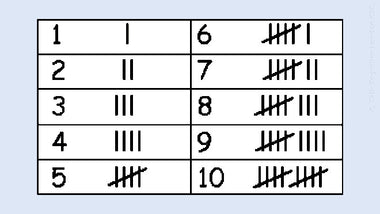Encourage children to make informal jottings or draw pictures to support their calculations. “Can you put something on paper to show that...?” Children begin to use symbols or marks rather than pictures: Dan spent 4p. What was his change from 10p?Children are taught to use a 100 square to subtract multiples of 10.

And here’s how division is taught in Key Stage 1:Introduce division to children in terms of sharing food fairly: for example, apples. How many apples are there for each child? Children share them equally: “one for you, one for me”.Use the language of division in every day contexts in class, for example, “There are 30 crayons and 6 children, how many crayons are there for each child?" The children can then practise sharing the objects out, one at a time.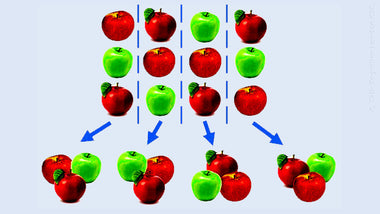After introducing division as sharing, teach how to understand division as grouping. For example: 12 ÷3. Children start with 12 objects and pull out groups of 3 at a time. “There is one group of 3, there is another group of 3” and so on. They then count how many groups they have.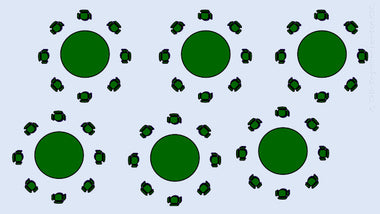Encourage them to solve problems by drawing. For example: 48 children sit at 6 tables. How many children are at each table if there are the same number at each? And, teach that division is the inverse (opposite) of multiplication. For example: 3 x 5 = 15 and 15 ÷ 5 = 3.Teach how to draw jumps on prepared number lines to help visualise division as repeated subtraction. For example, 15 ÷ 3. They then count the jumps to work out the answer.

Children who learn about all of these things about subtraction and division listed above then graduate to Key Stage 2.

Once they are in Key Stage 2, they are deemed to be ready for abstract thinking in order to apply the rules and algorithms of arithmetic and understand how and why they work.

### The importance of reasons over rules

Numeracy teachers' main role is to facilitate the progression from concrete resources to abstract learning of mathematical objects.In Key Stage 2, children are expected to have an abstract understanding of numbers and mathematical operations, which is independent of any physical object, image or thing:

To be able to subtract or divide without counting real objects or their images, children must develop the skill of abstract thinking and understand the meaning of the rules and algorithms.

For example, the process of ‘carrying over’ shown above in the example of adding 234 and 567 is abstracted from partitioning real objects into bundles of 10.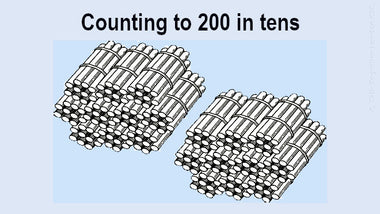These bundle pictures display concretely how to partition the number 200 into 2 bundles of hundreds, and each bundle of 100 into 10 bundles of tens.

Applying the concept of 'borrowing', used in the subtraction example above, depends on knowing how place value works. We need to partition the number 4085 in a similar way to be able to carry out the subtraction.

When subtracting 396 from 4085, we are decomposing 4085 into 'bundles' as 4 thousands, 0 hundreds, 8 tens and 5 units, according to place value, and only then 'borrowing' as needed and subtracting.

When dividing 7505 by 5, the answer is 1501 and not 151 because of the importance of zero as a place holder in the dividend 7505.

Rules and algorithms are introduced in Key Stage 2 because this is when children are expected to develop the ability to have a more abstract understanding of numbers and the four operations of arithmetic - addition, subtraction, multiplication and division.

### Conclusion: can talking overcome fear?

How can we support children to become more confident and fluent in mathematics? The key to both confidence and fluency is not in memorising one or two procedures and rules. It is in understading why they are doing what they are doing and to know when it is appropriate to use different methods.

An endless regime of practice with formal algorithms and rules does not guarantee that children may have success in constructing a good understanding of fundamental mathematical relationships and how they work.

However, knowing about place value and doing exercises that help them understand the relationships between the four operations of arithmetic will lead to the development of confidence and fluency with numbers.

It is as if when we do mathematics we want to be seen as omniscient because in the world of mathematics there is ‘right’ and ‘wrong’ and only one correct solution for each question. As grown-ups we feel the weight of this fact, even at the level of primary school arithmetic.

We are more fearful of making mistakes and being embarrassed in mathematics than in spelling or punctuation or grammar. And, as a result, many parents and carers will be reluctant to talk about the meaning of the rules of arithmetic or the reasons for the algorithms with their children.

We believe and hope that knowing the rules and applying the algorithms step by step will get us the right answers every time!

It probably happens more often than we'd like to think that too many adults emphasize rules and algorithms rather than understanding because they do not wish to make errors in finding the correct answers.

But, if we understand them, then we will not forget how the rules and algorithms work. It's easier to forget what to do or to be confused about solving a particular problem when their meaning was not understood in the first place.

Here is the catch: sometimes we will just be unable to apply the rules because we have forgotten or do not understand how and why they work, as in the examples given in the beginning of this post: 4085 - 396 = ? and 7505 ÷ 5 = ?

Talking about subtraction with 'borrowing' and long division with challenging dividends is going to help children - as well as parents and carers - understand how and why the rules work.

As for the experiences of parents and carers who are assisting with homework, if they know and understand the reasons, then it will be more fun to apply the rules they recall learning when they were students.

Sharing solution strategies, for example, can help begin a dialogue about how different rules work and encourage children to understand and appreciate mathematics more. Then, perhaps, everyone will have more fun studying mathematics.

(* Source: primaryresources.co.uk)